# Von Mangoldt function

This article defines an arithmetic function or number-theoretic function: a function from the natural numbers to a ring (usually, the ring of integers, rational numbers, real numbers, or complex numbers).
View a complete list of arithmetic functions

## Definition

### Hands-on definition

The von Mangoldt function, denoted, is an arithmetic function defined as follows:

•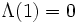.
•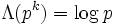, fora prime anda positive integer.
•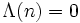ifhas more than one prime divisor.

### Definition in terms of Dirichlet product

The von Mangoldt function is the unique functionsuch that: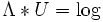whereis the logarithm,is the all ones function, anddenotes Dirichlet product.

By the Mobius inversion formula, this is equivalent to defining: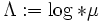,

whereis the Mobius function.